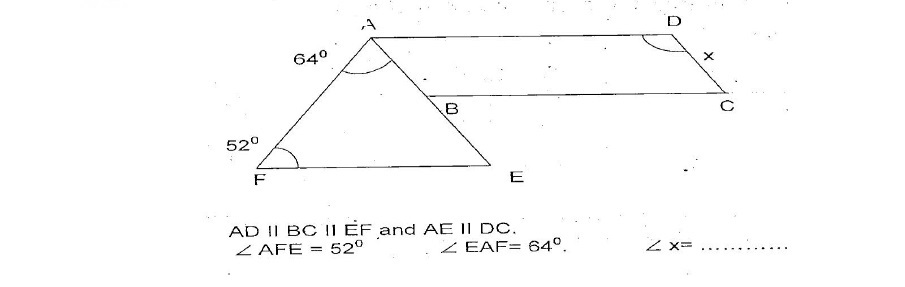# (Download) AISSEE Exam Paper - I Mathematics & Science "Class - IX"- 2016

Disclaimer: This website is NOT associated with CBSE, for official website of CBSE visit - www.cbse.gov.in## (Download) AISSEE Exam Paper - I Mathematics & Science "Class - IX"- 2016

ALL INDIA SAINIK SCHOOL ENTRANCE EXAMINATION: 2016
PAPER I – MATHEMATICS AND SCIENCE
CLASS –IX

Time: 2 hrs

Max Marks: 275
Notes:- (Maths: 200 & Science: 75)

(a) This paper contains two parts – Part ‘A’ is Mathematics and Part ‘B’ is Science. You are required to attempt ALL questions in each part.
(b) Part ‘A’ (Mathematics) contains 50 questions. Marks are indicated against each question.
(c) Part ‘B’ (Science) contains 37 questions. Marks are indicated against each question.
(d) Write your answers in the space provided in the question paper.
(e) Rough work is to be done at space provided only.
(f) Write all your answers in Blue/Black ink only. Do not use pencil.

This booklet contains 28 pages.

PART- A: MATHEMATICS (Max Marks: 200)
SECTOIN –I
(Each question carries two marks)

Q1. Find the product of (a2) x (2a22) x (4a26).
Q2. Using Euler’s formula find the number of faces of polyhedron having 6 vertices & 12 edges.
Q3. Calculate the value of 100 x 8 +10 x 1 + 7
Q4. What is the point of intersection of x-axis and y-axis called?
Q5. Find the value of x if
Q6. Eleven bags of wheat flour, each marked 5 kg, actually contained the following weights of flour (in kg) 4.97, 5.05, 5.08, 5.00, 5.06, 5.08, 4.98, 5.04, 5.07, 5.00. what is the probability that any of these bags chosen at contains
more than 5 kg of flour?
Q7. If X= [(56/28)0÷ (2/5 )3] x (16/25 ) then find the value of x.
Q8. Find cube root of 175616 by factorization method.
Q9. How many sides does a regular polygon have, if the measure of an exterior angle is 120?
Q10. Evaluate [(1/3)-1-(1/4)-1]-1